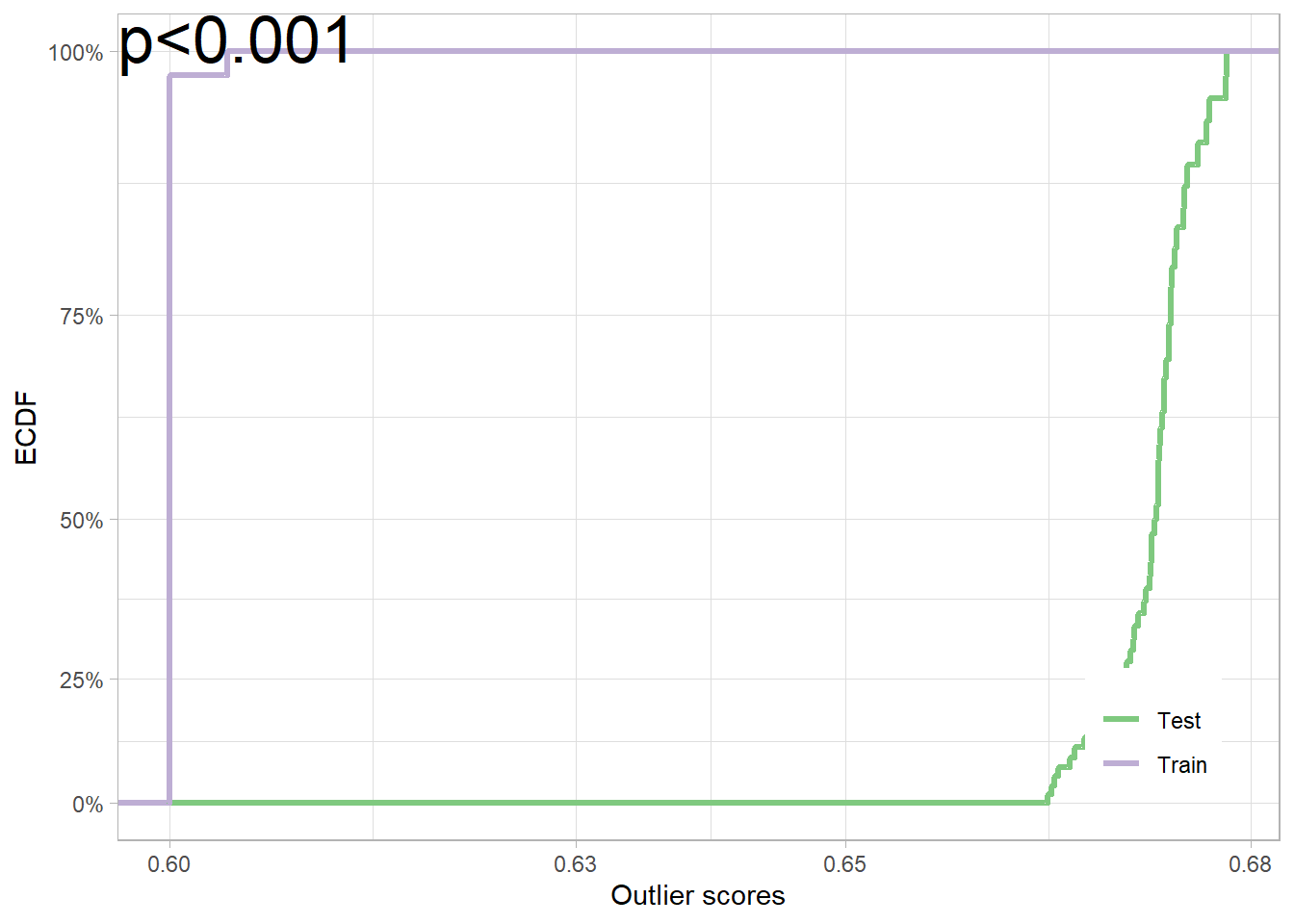Please see the paper as cited below (Kamulete 2022) for details. We denote the R package as dsos, to avoid confusion with D-SOS, the method.

## DIY

We show how easy it is to implement D-SOS for a particular notion of outlyingness. Suppose we want to test for no adverse shift based on isolation scores in the context of two-sample comparison. To do so, we need two main ingredients: a scoring function and a method to compute the $$p-$$value.

First, the scores are obtained using predictions from isolation forest with the isotree package (Cortes 2020). Isolation forest detects isolated points, instances that are typically out-of-distribution relative to the high-density regions of the data distribution. Naturally, any performant method for density-based out-of-distribution detection can effectively be used to achieve the same goal. The function score_od shows the implementation of one such scoring function in the dsos package.

dsos::score_od
## function (x_train, x_test, n_trees = 500L, threshold = 0.6)
## {
##     if (!requireNamespace("isotree", quietly = TRUE)) {
##         stop("Package \"isotree\" must be installed to use this function.",
##             call. = FALSE)
##     }
##     iso_fit <- isotree::isolation.forest(data = x_train, ntrees = n_trees)
##     os_train <- predict(iso_fit, newdata = x_train)
##     os_test <- predict(iso_fit, newdata = x_test)
##     os_train[os_train < threshold] <- threshold
##     os_test[os_test < threshold] <- threshold
##     return(list(test = os_test, train = os_train))
## }
## <bytecode: 0x0000013a865e5a30>
## <environment: namespace:dsos>

Second, we estimate the empirical null distribution for the $$p-$$value via permutations. For speed, this is implemented as a sequential Monte Carlo test with the simctest package (Gandy 2009). The function pt_refit in the dsos package combines scoring with inference. The prefix pt stands for permutation test. The code for _pt_ is relatively straightforward.

dsos may use sample splitting and out-of-bag variants as alternatives to permutations for $$p-$$value calculation. Both sample splitting and out-of-bag variants use the asymptotic null distribution for the test statistic. As a result, they can be appreciably faster than inference based on permutations.

dsos::pt_refit
## function (x_train, x_test, scorer, n_pt = 2000)
## {
##     result <- exchangeable_null(x_train, x_test, scorer = scorer,
##         n_pt = n_pt, is_oob = FALSE)
##     return(result)
## }
## <bytecode: 0x0000013a8679efa8>
## <environment: namespace:dsos>

## In Action

Take the iris dataset for example. When the training set only consists of setosa (flower species) and the test set, only of versicolor, the data is incompatible with the null of no adverse shift. In other words, we have strong evidence that the test contains a disproportionate number of outliers, if the training set is the reference distribution.

set.seed(12345)
data(iris)
x_train <- iris[1:50,1:4] # Training sample: Species == 'setosa'
x_test <- iris[51:100,1:4] # Test sample: Species == 'versicolor'
iris_test <- pt_refit(x_train, x_test, score = dsos::score_od)
plot(iris_test)You can plug in your own scores in this framework. Those already implemented in dsos can be useful but they are by means the only ones. If you favour a different method for out-of-distribution (outlier) detection, want to tune the hyperparameters, or choose a different notion of outlyingness altogether, dsos provides the building blocks to build your own. The workhorse function, powering the approach behind the scenes, is a way to calculate the test statistic from outlier scores (see wauc_from_os).